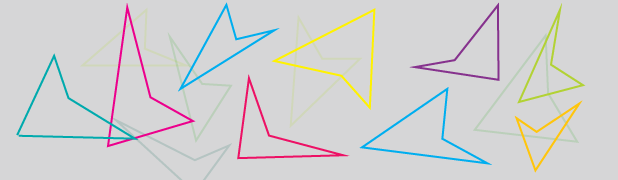The file attached with this problem contains a list having $100$ lines. Eight numbers $x_1, y_1, x_2, y_2, x_3, y_3, x_4, y_4$ are written in each line, which correspond to a quadrilateral whose vertices have coordinates $A_1=(x_1, y_1),\ A_2=(x_2, y_2),\ A_3=(x_3, y_3),\ A_4=(x_4, y_4)$ (in Cartesian plane). There are $N$ quadrilaterals in this list which are concave.

Find $N+6.$

Details and Assumptions:

• As an explicit example, suppose the list had $2$ lines, and the following numbers were written on those two lines: $\begin{array}{lrrrrrrrrl} &1, &1, &2, &2, &3, &3, &4, &4 \\ &1, &-1, &2, &-2, &3, &-3, &4, &-4 &.\end{array}$ We have two quadrilaterals, the first one having its vertices at points $(1, 1), (2, 2), (3, 3), (4, 4),$ and the second one having its vertices at points $(1, -1), (2, -2), (3, -3), (4, -4).$ Your job is to find out how many of them are concave.

• Link to the file: http://pastebin.ca/2679837.

• If one of the vertices lies on the line joining two other vertices, consider the resulting figure as convex.

• This is a computer science problem, and is inspired from ProjectEuler (I don't remember the problem number at the moment).

×